Home > CC4 > Chapter A > Lesson A.1.2 > ProblemA-23

A-23.

Without using a calculator, compute the value of each expression below. Homework Help ✎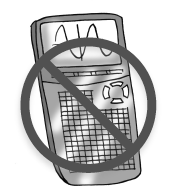1. 7 − 2 · (−5)

2. 6 + 3(7 − 3 · 2)2

3. 5 · (−3)2

4. 35 ÷ (16 − 32) · 2

5. −3 · 4 + 5 · (−2)

6. 7 − 6(10 − 4 · 2) ÷ 4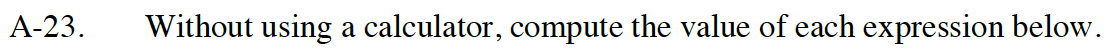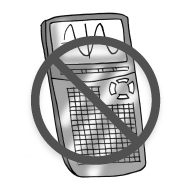Parentheses
Exponents
Multiplication and Division (left to right)
Addition and Subtraction (left to right)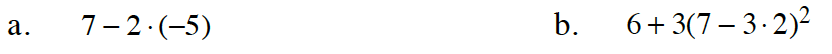7 − (−10)

17

The exponent applies only to what is inside the parentheses.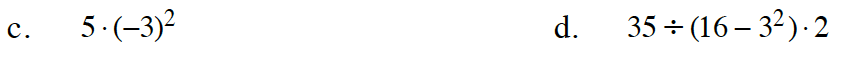45

See the order of operations.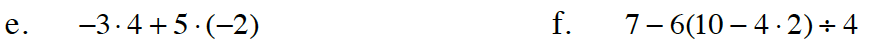See parts (a) through (d).

Do not confuse subtraction with a negative sign when multiplying.

4